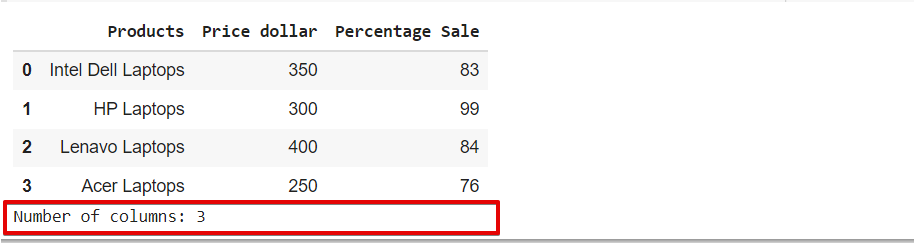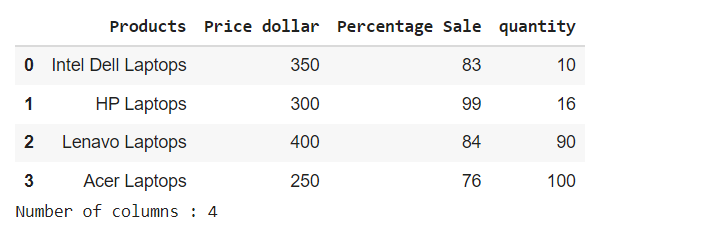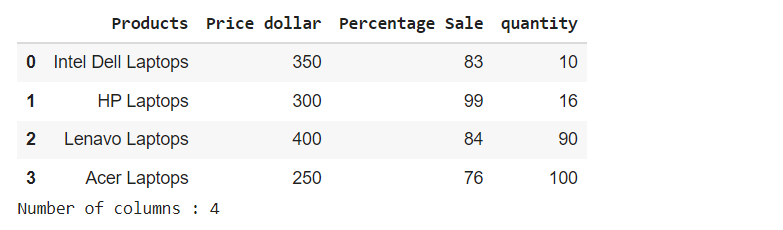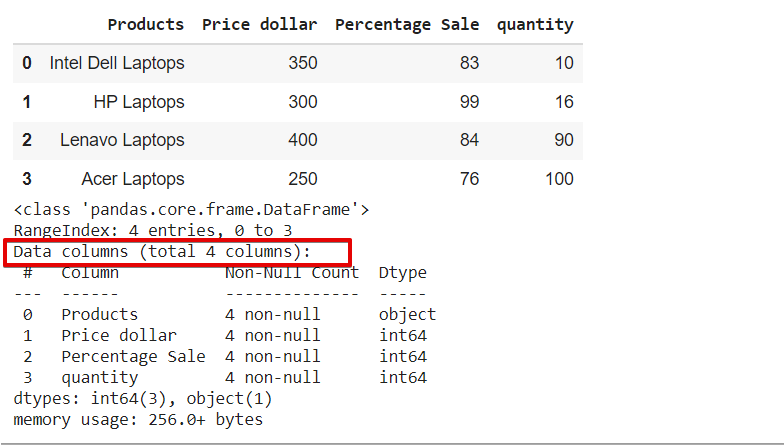# Count the Number of Pandas DataFrame Columns

Samreena Aslam Jan 30, 2023 Nov 03, 2021 Pandas Pandas DataFrame

In pandas `DataFrame`, data is stored or displayed in the tabular format like in `rows` and `columns`. Pandas help us in retrieving or counting the number of rows and columns in the `DataFrame` by using various approaches.

We will explore various methods in this tutorial related to counting the number of columns of a Pandas `DataFrame`.

## Count Number of Columns of Pandas `DataFrame` Using the `column` Property

Using the `column` property of the pandas `DataFrame`, we can retrieve the column list and calculate column length and count the number of columns in the `DataFrame`.

See the following example. First, we have created a `DataFrame` of products. Using `column_list = dataframe.columns` we retrieved the list of columns and then using `len(column_list)` counted the number of columns.

Example Codes:

``````import pandas as pd
import numpy as np
from IPython.display import display

# creating a DataFrame
dict = {'Products' : ['Intel Dell Laptops', 'HP Laptops', 'Lenavo Laptops', 'Acer Laptops'],
'Price dollar' : [350, 300, 400, 250 ],
'Percentage Sale' : [83, 99, 84, 76]}
dataframe = pd.DataFrame(dict)

# displaying the DataFrame
display(dataframe)

# To get the list of columns of dataframe
column_list = dataframe.columns

# Printing Number of columns
print('Number of columns:', len(column_list))
``````

Output:## Count Number of Columns of Pandas `DataFrame` Using the `shape` Property

It retrieves the tuples to represent the `DataFrame` shape when using the `shape` property. In the following example, the line `shape=dataframe.shape` will return the `DataFrame` shape and the `shape` counts the number of columns.

Example Codes:

``````import pandas as pd
import numpy as np
from IPython.display import display

# creating a DataFrame
dict = {'Products' : ['Intel Dell Laptops', 'HP Laptops', 'Lenavo Laptops', 'Acer Laptops'],
'Price dollar' : [350, 300, 400, 250 ],
'Percentage Sale' : [83, 99, 84, 76],
'quantity' : [10, 16, 90, 100]}
dataframe = pd.DataFrame(dict)

# displaying the DataFrame
display(dataframe)

# Get shape of the dataframe
shape = dataframe.shape

# Printing Number of columns
print('Number of columns :', shape)
``````

Output:As we can see in the above output, it displays the total number of columns is `4` in the above example.

## Count Number of Columns of Pandas `DataFrame` Using the Typecasting

We use the typecasting approach in this method, which is almost similar to the column property. When we use `typecasting` to the `DataFrame` list, it retrieves the list of column names. See the following example for more understanding about the typecasting approach:

Example Code:

``````import pandas as pd
import numpy as np
from IPython.display import display

# creating a DataFrame
dict = {'Products' : ['Intel Dell Laptops', 'HP Laptops', 'Lenavo Laptops', 'Acer Laptops'],
'Price dollar' : [350, 300, 400, 250 ],
'Percentage Sale' : [83, 99, 84, 76],
'quantity' : [10, 16, 90, 100]}
dataframe = pd.DataFrame(dict)

# displaying the DataFrame
display(dataframe)

# Typecasting dataframe to list
dataframe_list = list(dataframe)

# Printing Number of columns
print('Number of columns :', len(dataframe_list))
``````

Output:## Count the Number of Columns of Pandas `DataFrame` Using `dataframe.info()` Method

Using the `info()` method, we can print the complete concise summary of the pandas `DataFrame`. In the following example, we used `dataframe.info()` at the end of the source code. It displays the information related to `DataFrame` class, `dtypes`, memory usage, count number of columns, and ranges index.

Example Codes:

``````import pandas as pd
import numpy as np
from IPython.display import display

# creating a DataFrame
dict = {'Products' : ['Intel Dell Laptops', 'HP Laptops', 'Lenavo Laptops', 'Acer Laptops'],
'Price dollar' : [350, 300, 400, 250 ],
'Percentage Sale' : [83, 99, 84, 76],
'quantity' : [10, 16, 90, 100]}
dataframe = pd.DataFrame(dict)

# displaying the DataFrame
display(dataframe)

# Print dataframe information using info() method
dataframe.info()

``````

Output:In the above image, we can see the concise summary of the `DataFrame`, including the columns count.

## Related Article - Pandas DataFrame

• Get Pandas DataFrame Column Headers as a List
• Delete Pandas DataFrame Column
• Convert Pandas Column to Datetime
• Convert a Float to an Integer in Pandas DataFrame
• Sort Pandas DataFrame by One Column's Values
• Get the Aggregate of Pandas Group-By and Sum